# 如何基于HTML和CSV报表可视化多币种交易历史

24 五月 2019, 08:57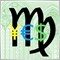0
2 088

### SubChart 指标

`(ENUM_CHART_MODE)ChartGetInteger(0, CHART_MODE)`

ENUM_CHART_MODE 枚举中包含了相同目标的元素: CHART_CANDLES, CHART_BARS, CHART_LINE.

```#property indicator_separate_window
#property indicator_buffers 4
#property indicator_plots   1
```

```input string SubSymbol = ""; // 交易品种
input bool Exact = false;
```

Exact 参数确定主窗口中的柱没有完全相同时间的另一个交易品种的匹配柱的情况下的操作。这个参数将在 iBarShift 函数调用中使用，其视觉效果如下：

• 如果“Exact” 等于 “false”，则函数返回最接近指定时间的最接近合适的柱数，因此如果没有报价（由于假日或其他原因），则指标将显示上一个柱；
• 如果 Exact = true, 函数返回 -1, 这样的画，指标图表在这个位置将是空白;

SubSymbol 参数默认等于空字符串，这表示报价与主窗口中显示的是一样的。在这种情况下，您应当编辑实际变量的值，把它设为 _Symbol. 视觉测试但是，由于“输入参数”是MQL中的只读变量，因此我们必须输入中间变量“Symbol”，并将其填充到OnInit处理程序中。

```string Symbol;

int OnInit()
{
Symbol = SubSymbol;
if(Symbol == "") Symbol = _Symbol;
else SymbolSelect(Symbol, true);
...
```

```ENUM_CHART_MODE mode = 0;
```

```// OHLC
double open[];
double high[];
double low[];
double close[];
```

```void InitBuffer(int index, double &buffer[], ENUM_INDEXBUFFER_TYPE style)
{
SetIndexBuffer(index, buffer, style);
ArraySetAsSeries(buffer, true);
}
```

```void InitPlot(int index, string name, int style, int width = -1, int colorx = -1)
{
PlotIndexSetInteger(index, PLOT_DRAW_TYPE, style);
PlotIndexSetDouble(index, PLOT_EMPTY_VALUE, 0);
PlotIndexSetString(index, PLOT_LABEL, name);
if(width != -1) PlotIndexSetInteger(index, PLOT_LINE_WIDTH, width);
if(colorx != -1) PlotIndexSetInteger(index, PLOT_LINE_COLOR, colorx);
}
```

```int Mode2Style(/*global ENUM_CHART_MODE mode*/)
{
switch(mode)
{
case CHART_CANDLES: return DRAW_CANDLES;
case CHART_BARS: return DRAW_BARS;
case CHART_LINE: return DRAW_LINE;
}
return 0;
}
```

```  InitBuffer(0, open, INDICATOR_DATA);
string title = "# Open;# High;# Low;# Close";
StringReplace(title, "#", Symbol);
mode = (ENUM_CHART_MODE)ChartGetInteger(0, CHART_MODE);
InitPlot(0, title, Mode2Style());

InitBuffer(1, high, INDICATOR_DATA);
InitBuffer(2, low, INDICATOR_DATA);
InitBuffer(3, close, INDICATOR_DATA);
```

```void SetPlotColors()
{
if(mode == CHART_CANDLES)
{
PlotIndexSetInteger(0, PLOT_COLOR_INDEXES, 3);
PlotIndexSetInteger(0, PLOT_LINE_COLOR, 0, (int)ChartGetInteger(0, CHART_COLOR_CHART_LINE));  // 长方形
PlotIndexSetInteger(0, PLOT_LINE_COLOR, 1, (int)ChartGetInteger(0, CHART_COLOR_CANDLE_BULL)); // 向上
PlotIndexSetInteger(0, PLOT_LINE_COLOR, 2, (int)ChartGetInteger(0, CHART_COLOR_CANDLE_BEAR)); // 向下
}
else
{
PlotIndexSetInteger(0, PLOT_COLOR_INDEXES, 1);
PlotIndexSetInteger(0, PLOT_LINE_COLOR, (int)ChartGetInteger(0, CHART_COLOR_CHART_LINE));
}
}
```

```  SetPlotColors();

IndicatorSetString(INDICATOR_SHORTNAME, "SubChart (" + Symbol + ")");
IndicatorSetInteger(INDICATOR_DIGITS, (int)SymbolInfoInteger(Symbol, SYMBOL_DIGITS));

return INIT_SUCCEEDED;
}
```

```void OnChartEvent(const int id,
const long& lparam,
const double& dparam,
const string& sparam)
{
if(id == CHARTEVENT_CHART_CHANGE)
{
mode = (ENUM_CHART_MODE)ChartGetInteger(0, CHART_MODE);
PlotIndexSetInteger(0, PLOT_DRAW_TYPE, Mode2Style());
SetPlotColors();
ChartRedraw();
}
}
```

```int OnCalculate(const int rates_total,
const int prev_calculated,
const datetime& time[],
const double& op[],
const double& hi[],
const double& lo[],
const double& cl[],
const long& tick_volume[],
const long& volume[],
{
static int lastAvailable = 0;
static bool initialized = false;

int _prev_calculated = prev_calculated;

if(iBars(Symbol, _Period) - lastAvailable > 1) // 填充柱的间隙
{
_prev_calculated = 0;
lastAvailable = 0;
initialized = false;
}

if(_prev_calculated == 0)
{
for(int i = 0; i < rates_total; ++i)
{
open[i] = 0;
high[i] = 0;
low[i] = 0;
close[i] = 0;
}
}
```

```  if(_Symbol != Symbol)
{
for(int i = 0; i < MathMax(rates_total - _prev_calculated, 1); ++i)
{
datetime dt = iTime(_Symbol, _Period, i);
int x = iBarShift(Symbol, _Period, dt, Exact);
if(x != -1)
{
open[i] = iOpen(Symbol, _Period, x);
high[i] = iHigh(Symbol, _Period, x);
low[i] = iLow(Symbol, _Period, x);
close[i] = iClose(Symbol, _Period, x);
}
}
}
```

```  else
{
ArraySetAsSeries(op, true);
ArraySetAsSeries(hi, true);
ArraySetAsSeries(lo, true);
ArraySetAsSeries(cl, true);
for(int i = 0; i < MathMax(rates_total - _prev_calculated, 1); ++i)
{
open[i] = op[i];
high[i] = hi[i];
low[i] = lo[i];
close[i] = cl[i];
}
}
```

initialized”静态变量包含更新完成的交易品种，如果 RefreshHistory 返回成功的标志，或者第三方交易品种柱的数量保持不变且非零（如果所需柱数没有历史记录），则将其设置为true。

```  if(lastAvailable == iBars(Symbol, _Period) && lastAvailable != 0)
{
if(!initialized)
{
Print("Updated ", Symbol, " ", iBars(Symbol, _Period), " bars");
initialized = true;
}
return rates_total;
}

if(!initialized)
{
if(_Symbol != Symbol)
{
Print("Updating ", Symbol, " ", lastAvailable, " -> ", iBars(Symbol, _Period), " bars up to ", (string)time, "... Please wait");
int result = RefreshHistory(Symbol, time);
if(result >= 0 && result <= 2)
{
_prev_calculated = rates_total;
}
if(result >= 0)
{
initialized = true;
ChartSetSymbolPeriod(0, _Symbol, _Period);
}
}
else
{
initialized = true;
}
}

lastAvailable = iBars(Symbol, _Period);

return _Symbol != Symbol ? _prev_calculated : rates_total;
}
```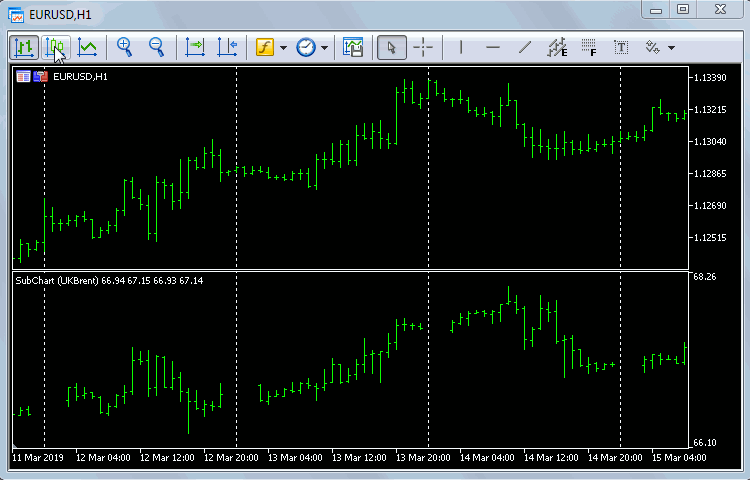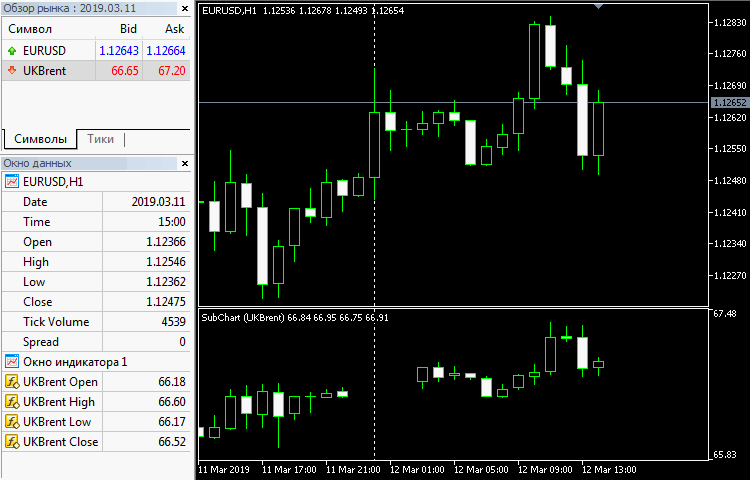### SubChartReporter 指标

```input string ReportFile = ""; // · ReportFile
input string Prefix = ""; // · Prefix
input string Suffix = ""; // · Suffix
input int  TimeShift = 0; // · TimeShift
```

SubchartReporter 指标中的特殊类将处理数据的接收和图形对象（趋势线）的生成。

Processor 中描述了以下变量：

```class Processor
{
protected:
string symbol;
string realsymbol;
IndexMap *data;
ulong timestamp;
string prefix;
```
• symbol — 报告中当前工作交易品种的名称；
• realsymbol — 实际可用工作交易品种的名称，由于前缀和后缀的原因，可能与第三方报告中使用的交易品种不同；
• data — 一个交易操作数组（indexmap类在文章中已经很熟悉，它是从具有相同名称的头文件连接的）；
• timestamp and prefix — 辅助变量，用于将由指标生成的图表上图形对象的唯一命名；

```    string pressed;
```

Processor 类中实现了以下用于对象生成的辅助方法：

```    void createTrend(const long dealIn, const long dealOut, const int type, const datetime time1, const double price1, const datetime time2, const double price2, const string &description)
void createButton(const int x, const int y, const int dx, const int dy, const string text, const bool selected)
void controlPanel(const IndexMap &symbols)
```

createTrend 为单独的交易（趋势线和两个箭头）创建可视化表示，createButton 创建一个工作交易品种按钮，controlPanel 是报告中显示的所有符号的完整按钮集。按钮显示在子窗口的左下角。

Processor 类的公共接口包括两组方法：

• 虚拟方法，受子类中特定实现的约束；
• 非虚拟方法，提供单一标准功能；

```    virtual IndexMap *load(const string file) = 0;
virtual int getColumnCount() = 0;
virtual int getSymbolColumn() = 0;
virtual datetime getStart() = 0;
virtual bool applyInit() { return true; }
virtual void makeTrade(IndexMap *row) = 0;
virtual int render() = 0;

bool attach(const string file)
bool apply(const string _s = NULL)
bool isEmpty() const
string findrealsymbol()
string _symbol() const
string _realsymbol() const
void onChartEvent(const int id, const long& lparam, const double& dparam, const string& sparam)
```

• getColumnCount — 返回数据表中的列数
• getSymbolColumn — 返回具有交易操作交易品种名称的列的索引
• getStart — 返回第一个交易操作的日期
• applyInit — 处理前可选初始化内部数据结构的方法
• render — 基于内部数据结构中记录的图形对象生成方法

```    bool attach(const string file)
{

IndexMap symbols;

for(int i = 0; i < data.getSize(); ++i)
{
IndexMap *row = data[i];
// 收集唯一的交易品种
string s = row[getSymbolColumn()].get<string>();
StringTrimLeft(s);
if(StringLen(s) > 0) symbols.set(s);
}

if(symbols.getSize() > 0)
{
controlPanel(symbols);
}
return true;
}
```

```
bool apply(const string _s = NULL)
{
ObjectsDeleteAll(0, "SCR", ChartWindowFind(), OBJ_TREND);

if(_s != NULL && _s != "") symbol = _s;
if(symbol == NULL)
{
Print("没有选择交易品种");
return false;
}

string real = findrealsymbol();
if(real == NULL)
{
Print("没有找到合适的交易品种");
return false;
}

SymbolSelect(real, true);

if(!applyInit()) return false;

int selected = 0;

for(int i = 0; i < data.getSize(); ++i)
{
IndexMap *row = data[i];

string s = row[getSymbolColumn()].get<string>();
StringTrimLeft(s);

if(s == symbol)
{
selected++;
}
}

pressed = prefix + "#" + symbol;
ObjectSetInteger(0, pressed, OBJPROP_BGCOLOR, clrGreen);

int trends = render();
Print(data.getSize(), " records in total");
Print(selected, " trades for ", symbol);

string title = CHART_REPORTER_TITLE + " (" + symbol + ", " + (string)selected + " records, " + (string)trends + " trades)";
IndicatorSetString(INDICATOR_SHORTNAME, title);

ChartRedraw();
return true;
}
```

findrealsymbol 方法使用多种方法来搜索匹配的交易品种，它检查交易品种的市场数据（投标价格）的可用性，如果找到数据，则认为该交易品种是真实的。如果没有市场数据，程序将尝试匹配后缀和/或前缀参数（如果指定了后缀或前缀），即从交易品种名称中添加或删除它们。如果修改后收到价格，则表示已找到交易品种的有效别名。

_symbol 和 _realsymbol 方法从报告中返回当前符号的名称，并为您的帐户返回此交易品种或其有效副本。在分析您自己的报告时，您将收到相同的交易品种名称，除非经纪商排除了您的交易品种。

onChartEvent 方法处理 OnChartEvent, 也就是按钮点击事件。上一个选择的按钮（如果有）将像所有其他按钮一样变成灰色。重要的部分是对虚拟“apply”方法的调用，将从按钮标识符中提取的新交易品种的名称传递给该方法。

```    void onChartEvent(const int id, const long& lparam, const double& dparam, const string& sparam)
{
if(id == CHARTEVENT_OBJECT_CLICK)
{
int x = StringFind(sparam, "_#");
if(x != -1)
{
string s = StringSubstr(sparam, x + 2);
Print(s, " ", sparam, " ", pressed);

ObjectSetInteger(0, sparam, OBJPROP_STATE, false);
ObjectSetInteger(0, pressed, OBJPROP_STATE, false);
ObjectSetInteger(0, pressed, OBJPROP_BGCOLOR, clrGray);
pressed = "";

if(apply(s)) // 将设置按下状态和其它属性
{
ChartSetSymbolPeriod(0, _Symbol, _Period);
}
}
}
}
```

HTML报表分析所需的所有设置（如 RowSelector 和 ColumnSettingsFile）都已在头文件中指定。但它们可以被编辑，因为它们被描述为输入参数。默认参数适用于MetaTrader 5报告，从中分析交易表，并根据这些交易计算进一步显示的头寸。每个交易都由特殊“Deal”类的一个实例描述。“Deal”构造函数从报表表接收一个包含在 IndexMap中的条目。以下字段存储在“Deal”中：交易时间和价格、交易类型和方向、交易量和其他属性。

```class ReportProcessor: public Processor
{
private:
class Deal   //  如果 MQL5 可以支持类的私有访问设置，
{            // 交易将无法从外部世界获得，因此
public:    // 把栏位变为共有，就可以从 Processor 中直接访问了。
datetime time;
double price;
int type;      // +1 - buy, -1 - sell
int direction; // +1 - in, -1 - out, 0 - in/out
double volume;
double profit;
long deal;
long order;
string comment;

public:
Deal(const IndexMap *row) // 这是 MT5 交易
{
time = StringToTime(row[COLUMN_TIME].get<string>()) + TimeShift;
price = StringToDouble(row[COLUMN_PRICE].get<string>());
string t = row[COLUMN_TYPE].get<string>();
type = t == "buy" ? +1 : (t == "sell" ? -1 : 0);
t = row[COLUMN_DIRECTION].get<string>();
direction = 0;
if(StringFind(t, "in") > -1) ++direction;
if(StringFind(t, "out") > -1) --direction;
volume = StringToDouble(row[COLUMN_VOLUME].get<string>());
t = row[COLUMN_PROFIT].get<string>();
StringReplace(t, " ", "");
profit = StringToDouble(t);
deal = StringToInteger(row[COLUMN_DEAL].get<string>());
order = StringToInteger(row[COLUMN_ORDER].get<string>());
comment = row[COLUMN_COMMENT].get<string>();
}

bool isIn() const
{
return direction >= 0;
}

bool isOut() const
{
return direction <= 0;
}

bool isOpposite(const Deal *t) const
{
return type * t.type < 0;
}

bool isActive() const
{
return volume > 0;
}
};
```

```    RubbArray<Deal *> array;
RubbArray<Deal *> queue;
```

RubbArray 类是动态数组的包装器，动态数组自动扩展以适应传入的数据。

```    virtual IndexMap *load(const string file) override
{
return HTMLConverter::convertReport2Map(file, true);
}

virtual int getColumnCount() override
{
return COLUMNS_COUNT;
}

virtual int getSymbolColumn() override
{
return COLUMN_SYMBOL;
}
```

```#define COLUMNS_COUNT 13
#define COLUMN_TIME 0
#define COLUMN_DEAL 1
#define COLUMN_SYMBOL 2
...
```

applyInit 方法清空 'array' 和 'queue' 数组。

```    virtual bool applyInit() override
{
((BaseArray<Deal *> *)&queue).clear();
array.clear();
return true;
}
```

```    virtual void makeTrade(IndexMap *row) override
{
array << new Deal(row);
}
```

```    virtual int render() override
{
int count = 0;

for(int i = 0; i < array.size(); ++i)
{
Deal *current = array[i];

if(!current.isActive()) continue;

if(current.isOut())
{
// 首先尝试完全匹配
for(int j = 0; j < queue.size(); ++j)
{
if(queue[j].isIn() && queue[j].isOpposite(current) && queue[j].volume == current.volume)
{
string description;
StringConcatenate(description, (float)queue[j].volume, "[", queue[j].deal, "/", queue[j].order, "-", current.deal, "/", current.order, "] ", (current.profit < 0 ? "-" : ""), current.profit, " ", current.comment);
createTrend(queue[j].deal, current.deal, queue[j].type, queue[j].time, queue[j].price, current.time, current.price, description);
current.volume = 0;
queue >> j; // 从队列中删除
++count;
break;
}
}

if(!current.isActive()) continue;

// second try to perform partial close
for(int j = 0; j < queue.size(); ++j)
{
if(queue[j].isIn() && queue[j].isOpposite(current))
{
string description;
if(current.volume >= queue[j].volume)
{
StringConcatenate(description, (float)queue[j].volume, "[", queue[j].deal, "/", queue[j].order, "-", current.deal, "/", current.order, "] ", (current.profit < 0 ? "-" : ""), current.profit, " ", current.comment);
createTrend(queue[j].deal, current.deal, queue[j].type, queue[j].time, queue[j].price, current.time, current.price, description);

current.volume -= queue[j].volume;
queue[j].volume = 0;
++count;
}
else
{
StringConcatenate(description, (float)current.volume, "[", queue[j].deal, "/", queue[j].order, "-", current.deal, "/", current.order, "] ", (current.profit < 0 ? "-" : ""), current.profit, " ", current.comment);
createTrend(queue[j].deal, current.deal, queue[j].type, queue[j].time, queue[j].price, current.time, current.price, description);

queue[j].volume -= current.volume;
current.volume = 0;
++count;
break;
}
}
}

// 从队列中清除非活动的部分
for(int j = queue.size() - 1; j >= 0; --j)
{
if(!queue[j].isActive())
{
queue >> j;
}
}
}

if(current.isActive()) // is _still_ active
{
if(current.isIn())
{
queue << current;
}
}
}

if(!isQueueEmpty())
{
Print("Warning: not all deals are processed (probably, open positions left).");
}

return count;
}
```

SubChartReporter的主要源代码继承自SubChart，我们使用它检查了子窗口中第三方报价的显示，所以让我们来谈谈新的片段。

```Processor *processor = NULL;

int OnInit()
{
if(StringFind(ReportFile, ".htm") > 0)
{
processor = new ReportProcessor();
}
else if(StringFind(ReportFile, ".csv") > 0)
{
processor = new HistoryProcessor();
}
string Symbol = SubSymbol;
if(Symbol == "") Symbol = _Symbol;
else SymbolSelect(Symbol, true);
processor.apply(Symbol);
...
}

void OnDeinit(const int reason)
{
if(processor != NULL) delete processor;
}
```

```void OnChartEvent(const int id,
const long& lparam,
const double& dparam,
const string& sparam)
{
if(id == CHARTEVENT_CHART_CHANGE)
{
... // 相同的代码
}
else
{
processor.onChartEvent(id, lparam, dparam, sparam);
}
}
```

OnCalculate处理程序中，我们需要跟踪分析交易品i那种在响应用户操作时发生更改的情况，之后执行完整的重新计算：

```  string Symbol = processor._realsymbol();
if(Symbol == NULL) Symbol = _Symbol;
if(lastSymbol != Symbol)
{
_prev_calculated = 0;
lastAvailable = 0;
initialized = false;
IndicatorSetInteger(INDICATOR_DIGITS, (int)SymbolInfoInteger(Symbol, SYMBOL_DIGITS));
}
```

```  if(lastAvailable == iBars(Symbol, _Period) && lastAvailable != 0)
{
if(!initialized)
{
Print("Updated ", Symbol, " ", iBars(Symbol, _Period), " bars");
initialized = true;
if(ReportFile != "") //
{                    //
EventSetTimer(1);  //
}                    //
}

return rates_total;
}
```

```void OnTimer()
{
EventKillTimer();

if(processor.isEmpty()) // 只载入一次文件
{
if(processor.attach(ReportFile))
{
}
else
{
}
}
}
```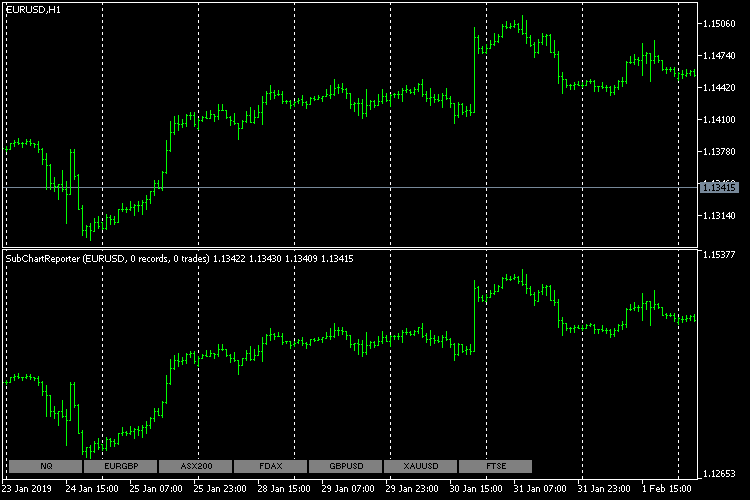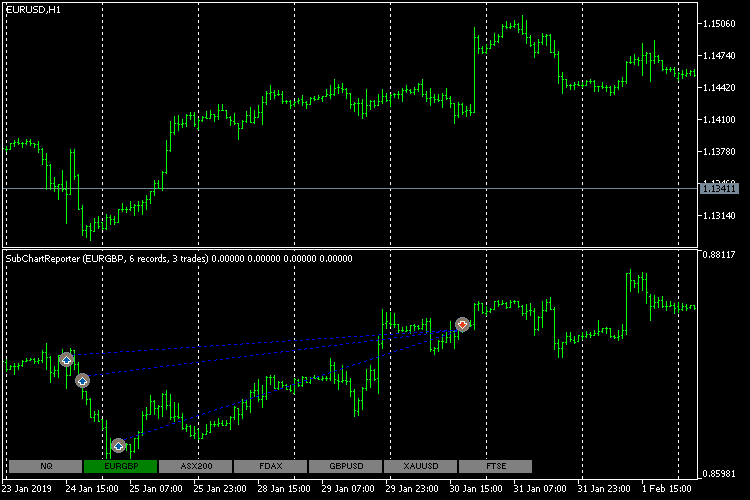### SubChartsBuilder 脚本

```bool createIndicator(const string symbol)
{
MqlParam params =
{
{TYPE_STRING, 0, 0.0, "::Indicators\\SubChartReporter.ex5"},

{TYPE_INT, 0, 0.0, NULL}, // 图表设置
{TYPE_STRING, 0, 0.0, "XYZ"},
{TYPE_BOOL, 1, 0.0, NULL},

{TYPE_INT, 0, 0.0, NULL}, // 通用设置
{TYPE_STRING, 0, 0.0, "HTMLCSV"},
{TYPE_STRING, 0, 0.0, "PREFIX"},
{TYPE_STRING, 0, 0.0, "SUFFIX"},
{TYPE_INT, 0, 0.0, NULL}, // 时间偏移

{TYPE_INT, 0, 0.0, NULL}, // html 设置
{TYPE_STRING, 0, 0.0, "ROW"},
{TYPE_STRING, 0, 0.0, "COLUMNS"},
{TYPE_STRING, 0, 0.0, "SUBST"},
{TYPE_BOOL, 0, 0.0, NULL},
{TYPE_BOOL, 0, 0.0, NULL},
{TYPE_BOOL, 0, 0.0, NULL},

{TYPE_INT, 0, 0.0, NULL}, // csv 设置
{TYPE_STRING, 0, 0.0, ";"}
};

params.string_value = symbol;
params.string_value = ReportFile;
params.string_value = Prefix;
params.string_value = Suffix;
params.integer_value = TimeShift;
params.string_value = RowSelector;
params.string_value = ColumnSettingsFile;
params.string_value = SubstitutionSettingsFile;
params.string_value = CSVDelimiter;

int handle = IndicatorCreate(_Symbol, _Period, IND_CUSTOM, 18, params);
if(handle == INVALID_HANDLE)
{
Print("无法创建 SubChartReporter  为  ", symbol, ": ", GetLastError());
return false;
}
else
{
{
Print("无法附加 SubChartReporter 为 ", symbol, ": ", GetLastError());
return false;
}
}
return true;
}
```

```#resource "\\Indicators\\SubChartReporter.ex5"
```

```int OnStart()
{
IndexMap *data = NULL;
int columnsCount = 0, symbolColumn = 0;

if(ReportFile == "")
{
Print("cleanUpChart");
return cleanUpChart();
}
else if(StringFind(ReportFile, ".htm") > 0)
{
data = HTMLConverter::convertReport2Map(ReportFile, true);
columnsCount = COLUMNS_COUNT;
symbolColumn = COLUMN_SYMBOL;
}
else if(StringFind(ReportFile, ".csv") > 0)
{
if(data != NULL && data.getSize() > 0)
{
IndexMap *row = data;
columnsCount = row.getSize();
symbolColumn = CSV_COLUMN_SYMBOL;
}
}

if(data != NULL)
{
IndexMap symbols;

for(int i = 0; i < data.getSize(); ++i)
{
IndexMap *row = data[i];
if(CheckPointer(row) == POINTER_INVALID || row.getSize() != columnsCount) break;

string s = row[symbolColumn].get<string>();
StringTrimLeft(s);
if(StringLen(s) > 0) symbols.set(s);
}

for(int i = 0; i < symbols.getSize(); ++i)
{
createIndicator(symbols.getKey(i));
}
delete data;
}

return 0;
}
```

```bool cleanUpChart()
{
bool result = true;
int n = (int)ChartGetInteger(0, CHART_WINDOWS_TOTAL);
for(int i = n - 1; i > 0; --i)
{
string name = ChartIndicatorName(0, i, 0);
if(StringFind(name, "SubChartReporter") == 0)
{
Print("Deleting ", name);
result &= ChartIndicatorDelete(0, i, name);
}
}
return result;
}
```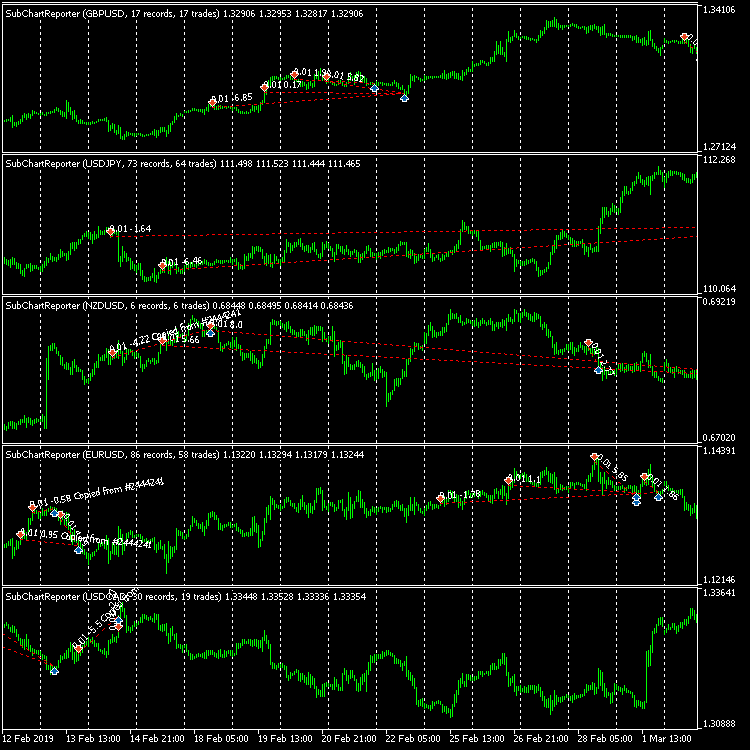### MainChartReporter 指标

Processor、ReportProcessor 和 HistoryProcessor 这三个主要类的源代码将移动到头文件中，并包含在这两个指标中。特定于任何版本的差异将在条件编译的预处理器指令中使用。CHART_REPORTER_SUB 宏将为 SubChartReporter指标代码定义，而 CHART_REPORTER_MAIN 宏将为 MainChartReporter定义。

```#ifdef CHART_REPORTER_MAIN
ChartSetSymbolPeriod(0, real, _Period);
#endif
```

```#ifdef CHART_REPORTER_MAIN
Comment(title);
#endif
```

```#ifdef CHART_REPORTER_SUB
ChartSetSymbolPeriod(0, _Symbol, _Period);
#endif
```

```#property indicator_chart_window
#property indicator_buffers 0
#property indicator_plots   0
```

```input GroupSettings Chart_Settings; // 子图表设置
input string SubSymbol = ""; // · 交易品种
input bool Exact = true; // · Exact
```

OnCalculate函数变为空，但它必须出现在指标中。从数据接收报告的计时器在OnInit中启动。

```void OnTimer()
{
EventKillTimer();

if(processor.isEmpty()) // 只载入一次文件
{
if(processor.attach(ReportFile))
{
processor.apply();
datetime start = processor.getStart();
if(start != 0)
{
ChartSetInteger(ChartID(), CHART_AUTOSCROLL, false);
// FIXME: 这里没有正确工作
ChartNavigate(ChartID(), CHART_END, -1 * (iBarShift(_Symbol, _Period, start)));
}
}
}
}
```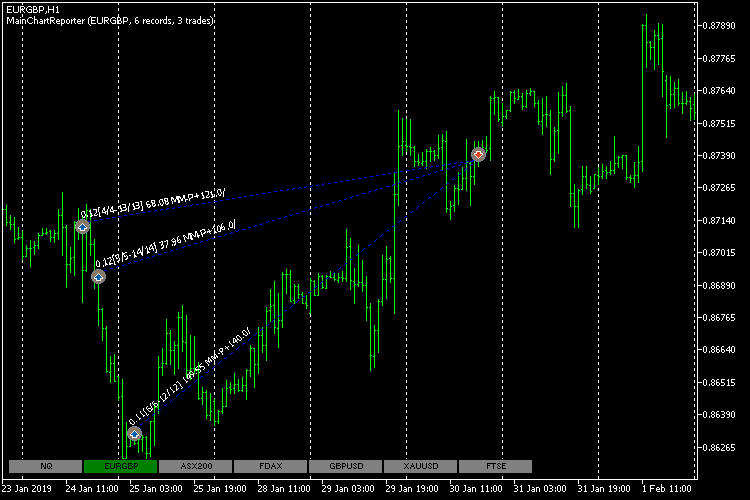MainChartReporter 指标

• SubChart.mq5 — SubChart 指标
• SubChartReporter.mq5 — SubChartReporter 指标
• MainChartReporter.mq5 — MainChartReporter 指标
• SubChartsBuilder.mq5 — 为报表中使用的所有交易品种创建一组SubchartReporter实例的脚本
• ChartReporterCore.mqh — 指标的主要共用类
• WebDataExtractor.mqh — HTML 解析器
• HTMLcolumns.mqh — 确定 HTML 报告列
• CSVcolumns.mqh — 确定 CSV 报告列
• IndexMap.mqh — 辅助映射类
• RubbArray.mqh — 辅助 rubber 数组类
• StringUtils.mqh — 字符串操作的工具函数
• empty_strings.h — HTML分析器的空标记列表
• GroupSettings.mqh — 空参数组
• Refresh.mqh — 请求交易品种报价
• ReportTester-example.html — HTML 测试报告实例
• ReportHistoryDeals.cfg.csv — 使用HTML分析器选择表列的CSS选择器设置

### 结论

report2chart.zip (34.55 KB)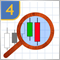研究烛条分析技术(第四部分)：形态分析器的更新和补充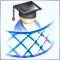开发一个跨平台网格 EA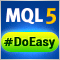轻松快捷开发 MetaTrader 程序的函数库(第四部分)：交易事件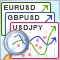利用 MQL5 和 MQL4 实现的选择和导航工具: 把数据添加到图表中